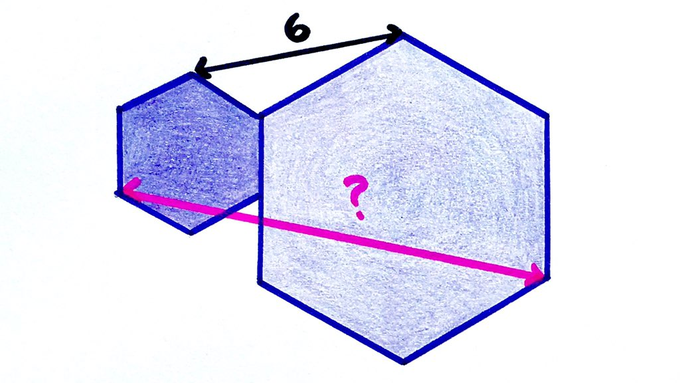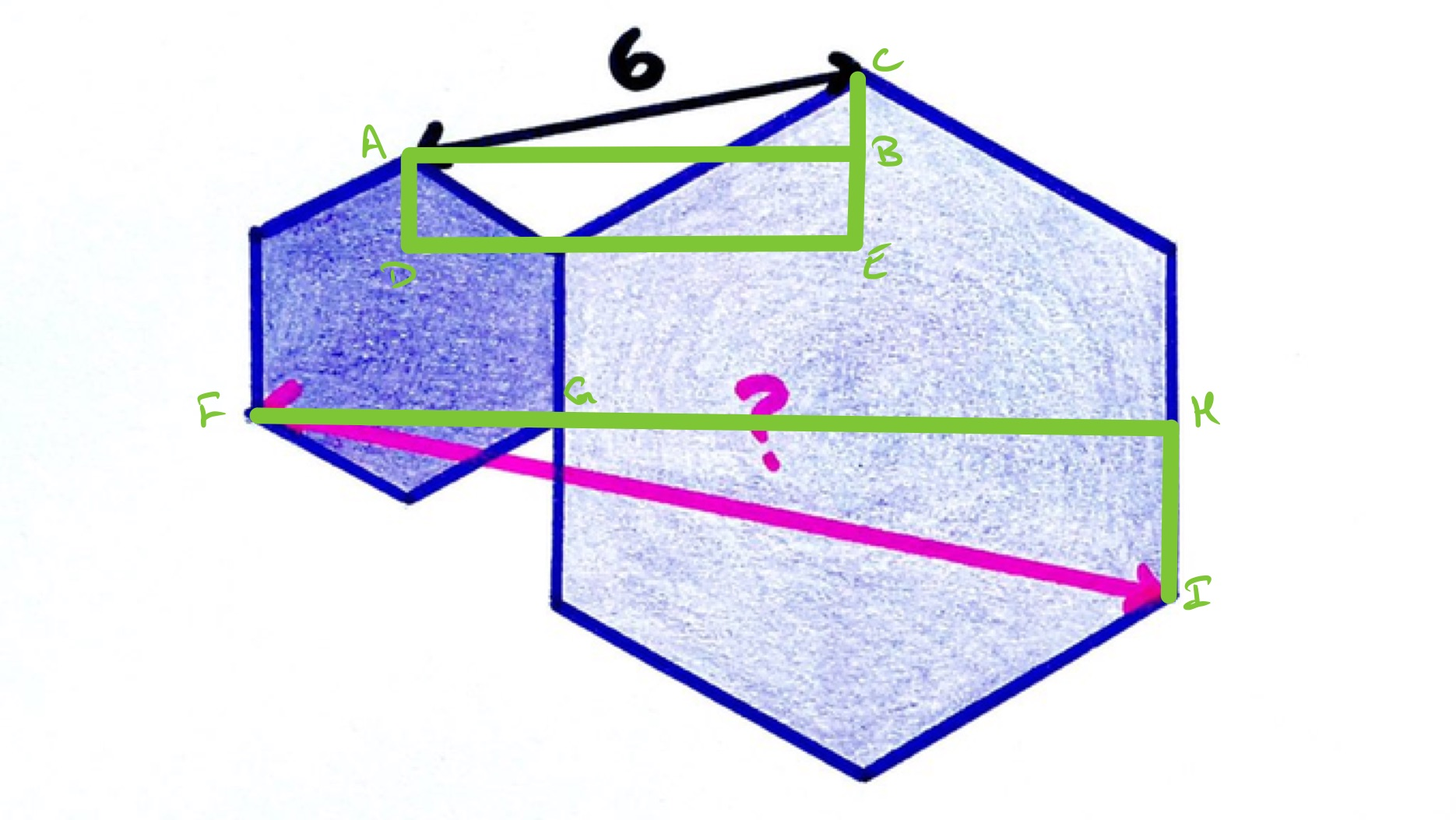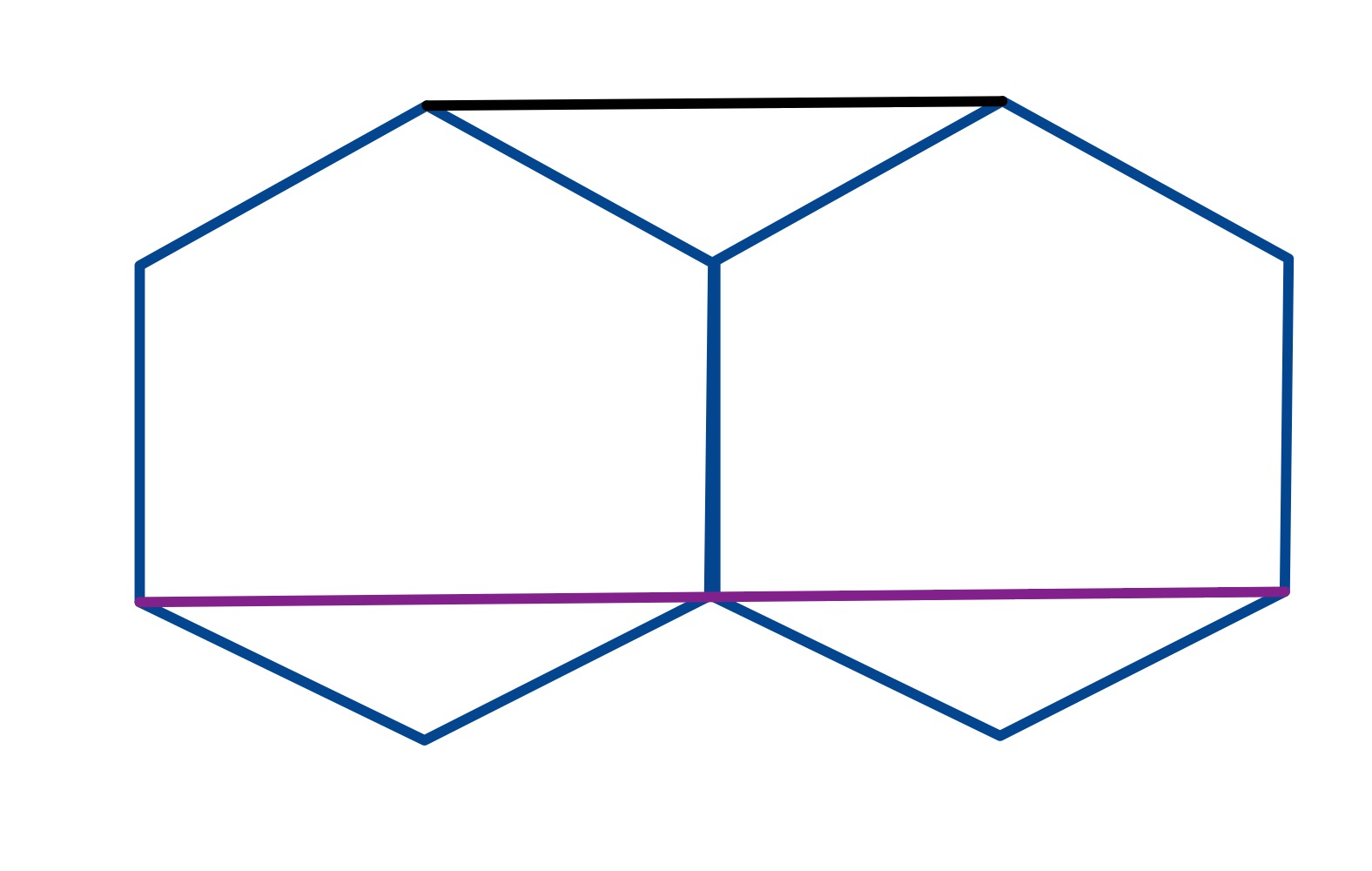# Solution to the Two Regular Hexagons (II) PuzzleBoth hexagons are regular. How long is the pink line?

## Solution by Lengths in a Regular Hexagon and Similar TrianglesConsider the triangles $A B C$ and $F H I$ in the diagram. The length of $A B$ is half the length of $F H$. The length of $I H$ is the difference of the sides. Using lengths in a regular hexagon, $A D$ and $C E$ have lengths half the side lengths of their respective hexagons, so the length of $C B$ is half the difference of these sides and so has half the length of $I H$. Since both $A B C$ and $F H I$ are right-angled triangles, this establishes them as similar with a scale factor of $2$. Therefore the length of $F I$ is twice that of $A C$ and so is of length $12$.

## Solution by Invariance PrincipleBy drawing the hexagons the same size, the relationship between the two lengths is clear and so the required length is $12$.